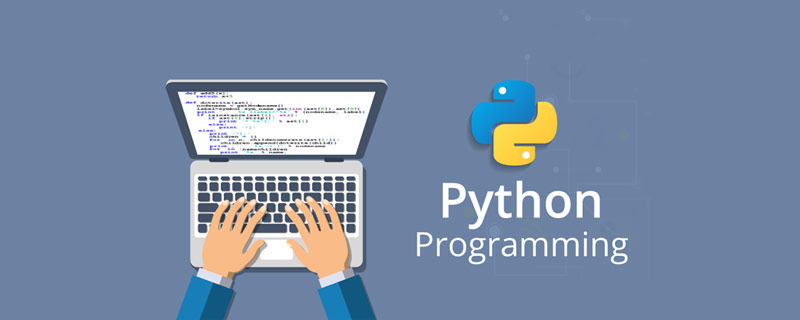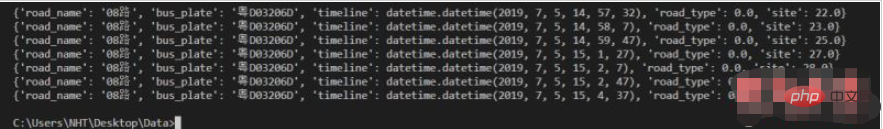# python如何读取excel表数据_编程技术_亿码酷站

python读取excel表数据的方法：首先安装Excel读取数据的库xlrd；然后获取Excel文件的位置并且读取进来；接着读取指定的行和列的内容，并将内容存储在列表中；最后运行程序即可。python读取excel表数据的方法：

1、安装Excel读取数据的库—–xlrd

```#引入Excel库的xlrd
import xlrd```

2、获取Excel文件的位置并且读取进来

```#导入需要读取Excel表格的路径
data = xlrd.open_workbook(r'C:\Users\NHT\Desktop\Data\\test1.xlsx')
table = data.sheets()```

3、读取指定的行和列的内容，并将内容存储在列表中（将第三列的时间格式转换）

```#创建一个空列表，存储Excel的数据
tables = []

#将excel表格内容导入到tables列表中
def import_excel(excel):
for rown in range(excel.nrows):
array['bus_plate'] = table.cell_value(rown,1)
#将Excel表格中的时间格式转化
if table.cell(rown,2).ctype == 3:
date = xldate_as_tuple(table.cell(rown,2).value,0)
array['timeline'] = datetime.datetime(*date)
array['site'] = table.cell_value(rown,4)
tables.append(array)```

4、运行程序

```if __name__ == '__main__':
#将excel表格的内容导入到列表中
import_excel(table)
#验证Excel文件存储到列表中的数据
for i in tables:
print(i)```

5、最终的运行效果如下：6、完整的程序代码：

```import xlrd
from xlrd import xldate_as_tuple
import datetime
#导入需要读取的第一个Excel表格的路径
data1 = xlrd.open_workbook(r'C:\Users\NHT\Desktop\Data\\test.xlsx')
table = data1.sheets()
#创建一个空列表，存储Excel的数据
tables = []
#将excel表格内容导入到tables列表中
def import_excel(excel):
for rown in range(excel.nrows):
array['bus_plate'] = table.cell_value(rown,1)
if table.cell(rown,2).ctype == 3:
date = xldate_as_tuple(table.cell(rown,2).value,0)
array['timeline'] = datetime.datetime(*date)
array['site'] = table.cell_value(rown,4)
tables.append(array)
if __name__ == '__main__':
#将excel表格的内容导入到列表中
import_excel(table)
for i in tables:
print(i)```

python如何读取excel表数据

php如何利用正则去掉注释

## Python语言支持编程方式有哪些？_亿码酷站_亿码酷站

Python语言支持编程方式有哪些？_亿码酷站_亿码酷站

## python如何实现颜色_编程技术_编程开发技术教程

python如何实现颜色_编程技术_编程开发技术教程